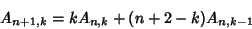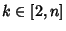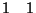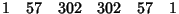## Euler's Triangle

The triangle of numbersgiven byand the Recurrence Relationfor, whereare Eulerian Numbers.The numbers 1, 1, 1, 1, 4, 1, 1, 11, 11, 1, ... are Sloane's A008292. Amazingly, the Z-Transform ofare generators for Euler's triangle.

See also Clark's Triangle, Eulerian Number, Leibniz Harmonic Triangle, Number Triangle, Pascal's Triangle, Seidel-Entringer-Arnold Triangle, Z-Transform

References

Sloane, N. J. A. Sequence A008292 in The On-Line Version of the Encyclopedia of Integer Sequences.'' http://www.research.att.com/~njas/sequences/eisonline.html.